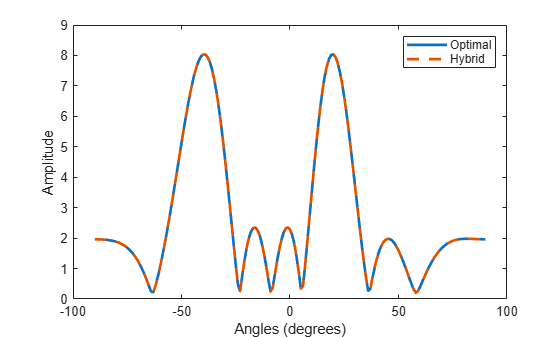# ompdecomp

Decompose signal using orthogonal matching pursuit

## Syntax

``[coeff,dictatom,atomidx,errnorm] = ompdecomp(X,dict)``
``[coeff,dictatom,atomidx,errnorm] = ompdecomp(X,dict,'MaxSparsity',nm)``
``[coeff,dictatom,atomidx,errnorm] = ompdecomp(X,dict,'NormWeight',wts)``

## Description

````[coeff,dictatom,atomidx,errnorm] = ompdecomp(X,dict)` computes the decomposition matrices `coeff` and `dictatom` of the signal `X`. The product of the decomposition matrices, `dictatom` x `coeff`, approximates `X`. The atoms in `dictatom` are selected from `dict`. `atomidx` are the indices in `dict` corresponding to `dictatom`. `errnorm` is the decomposition error. The decomposition is based on an orthogonal matching pursuit (OMP) algorithm that minimizes the Frobenius norm `||``X` – `dictatom` x `coeff``||`.```

example

````[coeff,dictatom,atomidx,errnorm] = ompdecomp(X,dict,'MaxSparsity',nm)` also specifies the maximum sparsity `nm`.```
````[coeff,dictatom,atomidx,errnorm] = ompdecomp(X,dict,'NormWeight',wts)` minimizes the weighted Frobenius norm `||``wts`1/2(`X` – `dictatom` x `coeff`)`||` using the weights `wts`.```

## Examples

collapse all

Given a set of optimal, full-digital, beamforming weights for an 8-element uniform linear array, decompose the weights into a product of analog and digital beamforming weights. Assume two RF chains. Show that the combined weights achieve a performance similar to the optimal weights.

Specify the optimal, full-digital, beamforming weights.

`wopt = steervec((0:7)*0.5,[20 -40]);`

Create a dictionary of steering vectors.

`stvdict = steervec((0:7)*0.5,-90:90);`

Perform the decomposition using OMP. Set the maximum sparsity to two.

`[wbb,wrf,wdictidx,normerr] = ompdecomp(wopt,stvdict,'MaxSparsity',2);`

Compare the beam patterns derived from the optimal weights and the hybrid weights. The plot shows that the decomposition of `wopt` into `wrf` and `wbb` is almost exact.

```plot(-90:90,abs(sum(wopt'*stvdict)),'-', ... -90:90,abs(sum((wrf*wbb)'*stvdict)),'--','LineWidth',2) xlabel('Angles (degrees)') ylabel('Amplitude') legend('Optimal','Hybrid')```## Input Arguments

collapse all

Input data to be decomposed, specified as a complex-valued N-by-Nc matrix.

Data Types: `double`
Complex Number Support: Yes

Dictionary of atoms, specified as a complex-valued matrix. The function uses a subset of atoms from the dictionary to construct the data.

Data Types: `double`
Complex Number Support: Yes

Maximum sparsity of the decomposition, specified as a positive integer. The decomposition stops when the sparsity of `nm` is achieved.

Example: `5`

#### Dependencies

Use this argument with the syntax specifying `'MaxSparsity'`.

Data Types: `double`

Norm weights used by OMP to minimize the weighted Frobenius norm of `||``wts`1/2 x (`X``dictatom` x `coeff`)`||`, specified as a complex-valued N-by-N matrix.

Example: `5`

#### Dependencies

Use this argument with the syntax specifying `'NormWeight'`.

Data Types: `double`
Complex Number Support: Yes

## Output Arguments

collapse all

Coefficients of basis atoms, returned as an Ns-by-Nc matrix. The rows represent the coefficients for the corresponding atoms in `dictatom`. Ns represents the number of atoms selected from the dictionary and is a measure of signal sparsity.

Data Types: `double`
Complex Number Support: Yes

Signal basis atoms, returned as an N-by-Ns matrix. The columns are the atoms forming the basis of the signal. These atoms are a subset of the dictionary specified in `dict`. Ns represents the number of selected atoms and is a measure of signal sparsity.

Data Types: `double`
Complex Number Support: Yes

Indices of the atoms selected from the dictionary `dict`, returned as a length-Ns row vector where `dict(:,atomidx) = dictatom`.

Data Types: `double`

Norm of the decomposition error, returned as a nonnegative scalar.

Data Types: `double`

collapse all

### Hybrid Beamforming Weights

In the context of hybrid beamforming, the `coeff` argument represents digital weights. `dictatom` represents analog weights and `dict` is a collection of steering vectors that can be used as analog weights.

 Ayach, Omar El et al. "Spatially Sparse Precoding in Millimeter Wave MIMO Systems" IEEE Trans on Wireless Communications. Vol. 13, No. 3, March 2014.